# 网格和马丁格尔：它们是什么？如何使用它们？

### 网格及其基本方程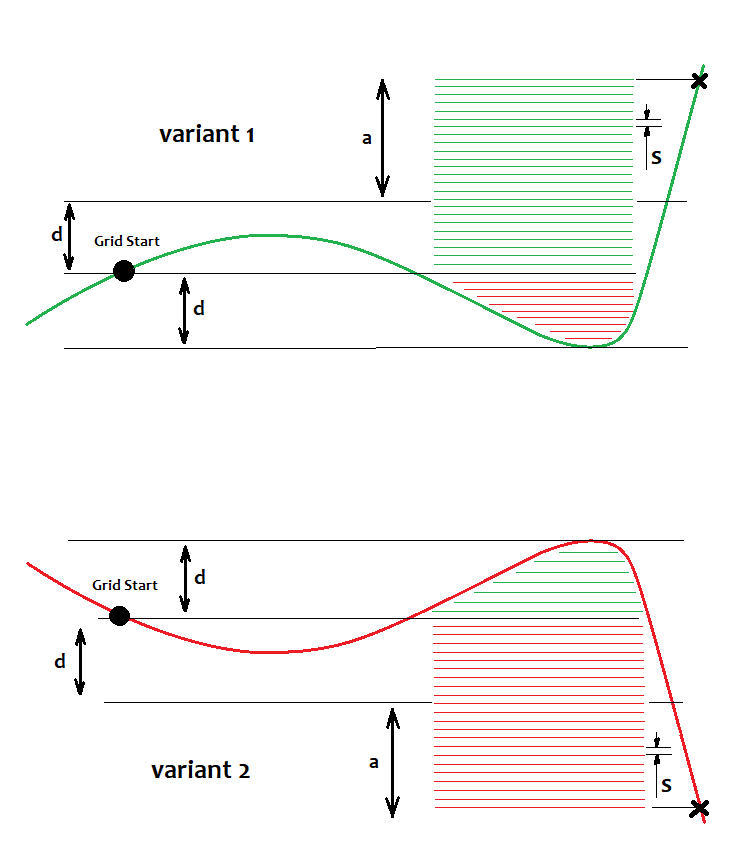• Nl=d/s - 1
• NP=(a+d)/s -1
• Pr=Sum(1,NP)(s*i)=(s+s*NP)*Np/2
• Ls=Sum(1,Nl)(s*j+ s*NP)=(s+s*Nl)*Nl/2+s*NP^2

• Prf=Pr/Ls
• M=(Pr-Ls)/(Np+Nl)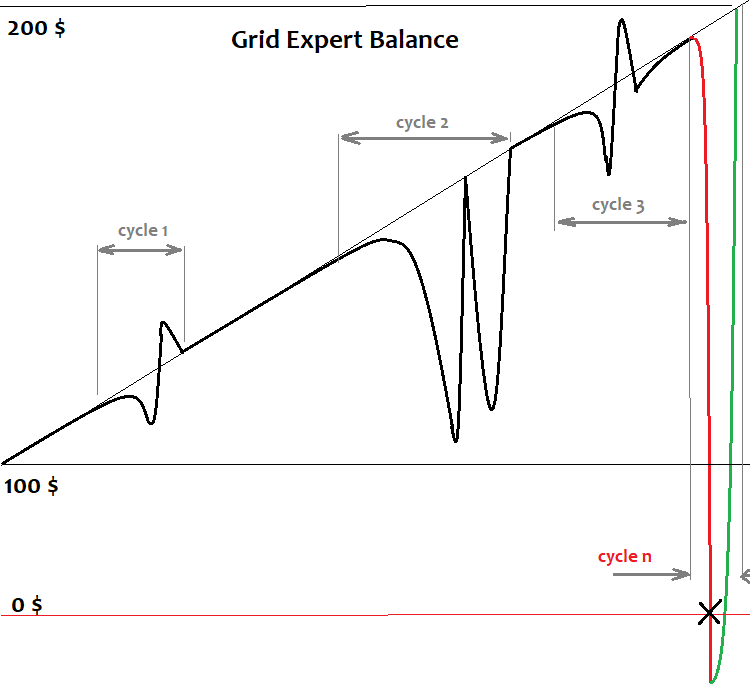### 马丁格尔和它的基本方程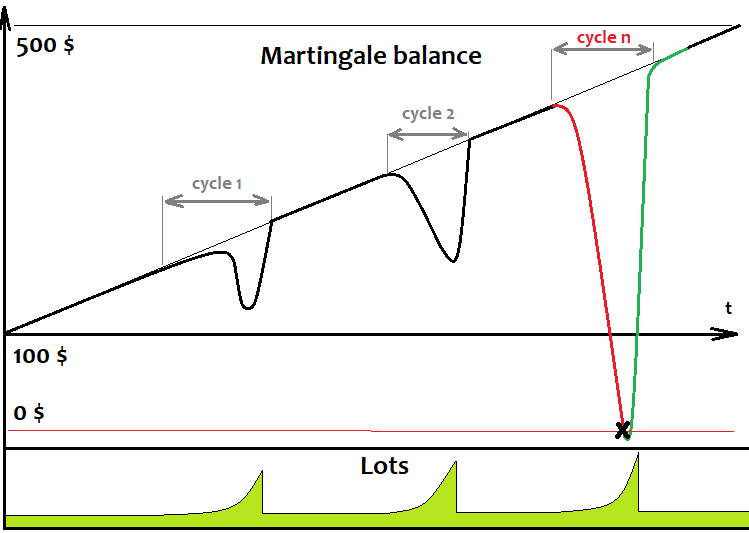• Nl
• Np=1
• Pr=L[Nl+Np]*TP[Nl+Np]*TickSize
• Ls=Sum(1,Nl)(L[i]*SL[i])*TickSize

• L=StartLot
• for(2,Nl) L[i]=(K*Sum(1,i-1)(L[j]*SL[j]))/TP[i]

### 开发和测试最简单的网格EA

```#include <Trade\PositionInfo.mqh>
```

```///grid variables
input int MaxChannelSizePoints=500;//Max Of a+d
input int MinMoveToClose=100;//Mininum Move
input int GridStepPoints=20;//Grid Step In Points
input int BarsI=999;//Bars To Start Calculate
input double KClose=3.5;//Asymmetry
///

input int SlippageMaxOpen=15; //Slippage For Open In Points
input double Lot=0.01;//Lot
input int MagicC=679034;//Magic
/////////
```

```void DimensionAllMQL5Values()//////////////////////////////
{
ArrayResize(Time,BarsI,0);
ArrayResize(High,BarsI,0);
ArrayResize(Low,BarsI,0);
}

void CalcAllMQL5Values()///////////////////////////////////
{
ArraySetAsSeries(High,false);
ArraySetAsSeries(Low,false);
ArraySetAsSeries(Time,false);
CopyHigh(_Symbol,_Period,0,BarsI,High);
CopyLow(_Symbol,_Period,0,BarsI,Low);
CopyTime(_Symbol,_Period,0,BarsI,Time);
ArraySetAsSeries(High,true);
ArraySetAsSeries(Low,true);
ArraySetAsSeries(Time,true);
}
```

```void RestoreGrid()//recover the grid if the robot is restarted
{
DimensionAllMQL5Values();
CalcAllMQL5Values();
bool ord=PositionSelect(Symbol());
if ( ord && int(PositionGetInteger(POSITION_MAGIC)) == MagicC )
{
GridStartTime=datetime(PositionGetInteger(POSITION_TIME));
GridStartPrice=double(PositionGetDouble(POSITION_PRICE_OPEN));
GridUpPrice=GridStartPrice;
GridDownPrice=GridStartPrice;

for(int i=0;i<BarsI;i++)
{
if ( High[i] > GridUpPrice ) GridUpPrice=High[i];
if ( Low[i] < GridDownPrice ) GridDownPrice=Low[i];
if ( Time[i] < GridStartTime ) break;
}
bCanUpdate=true;
}
}
```

```datetime GridStartTime;//grid construction time
double GridStartPrice;//grid starting price
double GridUpPrice;//upper price within the corridor
double GridDownPrice;//lower price within the corridor
```

```bool bCanUpdate;//whether it is possible to update the grid
bool bTryedAlready;//whether there was an attempt to close a position
```

```void CreateNewGrid()//create a new grid
{
SymbolInfoTick(Symbol(),LastTick);
GridStartTime=TimeCurrent();
GridStartPrice=LastTick.bid;
GridUpPrice=GridStartPrice;
GridDownPrice=GridStartPrice;

double SummDown=LastTick.bid-double(GridStepPoints)*_Point;

while ( SummUp <= LastTick.ask+double(MaxChannelSizePoints)*_Point )
{
SummUp+=double(GridStepPoints)*_Point;
}

while ( SummDown >= LastTick.bid-double(MaxChannelSizePoints)*_Point )
{
SummDown-=double(GridStepPoints)*_Point;
}
}

void UpdateGrid()//update the grid parameters
{
SymbolInfoTick(Symbol(),LastTick);
if ( LastTick.bid > GridUpPrice ) GridUpPrice=LastTick.bid;
if ( LastTick.bid < GridDownPrice ) GridDownPrice=LastTick.bid;
}
```

```void ClosePosition()//close a position by a symbol
{
bool ord;
ord=PositionSelect(Symbol());
if ( ord && int(PositionGetInteger(POSITION_MAGIC)) == MagicC  )
{
}
}

void CleanLimitOrders()//clear limit orders
{
int orders=OrdersTotal();
for(int i=0;i<orders;i++)
{
ulong ticket=OrderGetTicket(i);
if(ticket!=0)
{
}
}
}

bool bCanClose()//closure condition
{
if ( GridStartPrice == GridUpPrice && (GridStartPrice-GridDownPrice)/_Point >= MinMoveToClose ) return true;
if ( GridStartPrice == GridDownPrice && (GridUpPrice-GridStartPrice)/_Point >= MinMoveToClose ) return true;

if ( GridStartPrice != GridUpPrice && GridStartPrice != GridDownPrice
&& (GridStartPrice-GridDownPrice)/(GridUpPrice-GridStartPrice) >= KClose
&& (GridStartPrice-GridDownPrice)/_Point >= MinMoveToClose ) return true;
if ( GridStartPrice != GridDownPrice && GridStartPrice != GridUpPrice
&& (GridUpPrice-GridStartPrice)/(GridStartPrice-GridDownPrice) >= KClose
&& (GridUpPrice-GridStartPrice)/_Point >= MinMoveToClose ) return true;

/*
if ( GridUpPrice >= GridStartPrice+MaxChannelSizePoints*_Point
//|| GridDownPrice <= GridStartPrice-MaxChannelSizePoints*_Point ) return true;
*/
return false;
}
```

```void Trade()//the main function where all actions are performed
{
bool ord=PositionSelect(Symbol());

if ( bCanUpdate ) UpdateGrid();

if ( ord && bCanClose() )//if there is a position and the closing condition is met
{
ClosePosition();
CleanLimitOrders();
bCanUpdate=false;
}

if ( !bCanUpdate && !ord )
{
CleanLimitOrders();
CreateNewGrid();
bCanUpdate=true;
}
}
```

另外，让我们定义在何处、调用什么以及在初始化EA时执行什么操作：

```int OnInit()
{
m_trade.SetExpertMagicNumber(MagicC);//set the magic number for positions
RestoreGrid();//restore the grid if present
return(INIT_SUCCEEDED);
}

void OnTick()
{
}
```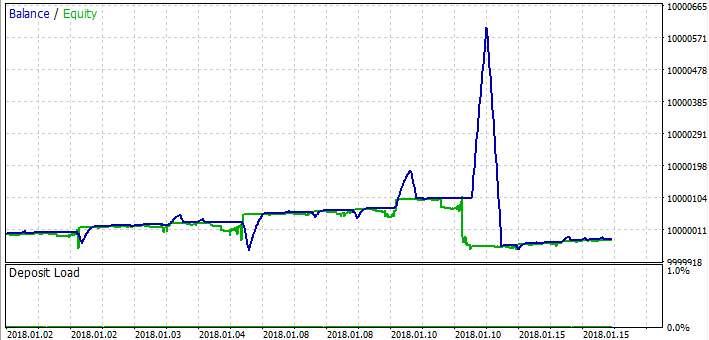### 开发和测试最简单的马丁格尔EA的

```input int SLE=100;//Stop Loss Points
input int TPE=300;//Take Profit Points
input int SlippageMaxOpen=15; //Slippage For Open In Points
input double Lot=0.01;//Start Lot
input int MagicC=679034;//Magic
input int HistoryDaysLoadI=10;//History Deals Window Days

```

```double CalcLot()//calculate the lot
{
bool ord;
double TotalLot=0;
for ( int i=HistoryDealsTotal()-1; i>=0; i-- )
{
ulong ticket=HistoryDealGetTicket(i);
ord=HistoryDealSelect(ticket);
if ( ord && HistoryDealGetString(ticket,DEAL_SYMBOL) == _Symbol
&& HistoryDealGetInteger(ticket,DEAL_MAGIC) == MagicC
&& HistoryDealGetInteger(ticket,DEAL_ENTRY) == DEAL_ENTRY_OUT )
{
if ( HistoryDealGetDouble(ticket,DEAL_PROFIT) < 0 )
{
TotalLot+=HistoryDealGetDouble(ticket,DEAL_VOLUME);
}
else
{
break;
}
}
}
}

void Trade()//the main function where all actions are performed
{
bool ord=PositionSelect(Symbol());
SymbolInfoTick(Symbol(),LastTick);
if ( !ord )
{
if ( MathRand() > 32767.0/2.0 )
{
}
else
{
}
}
}
```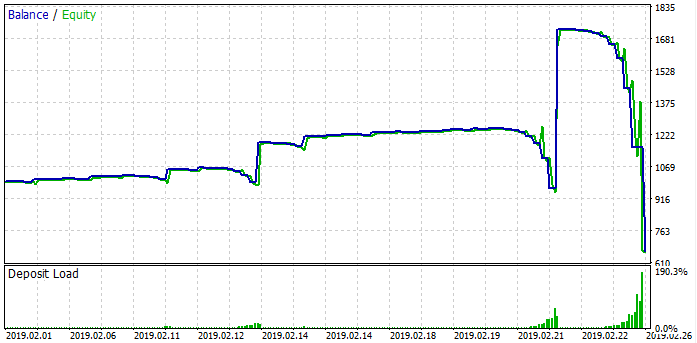### 网格和马丁格尔背后的统一数学原理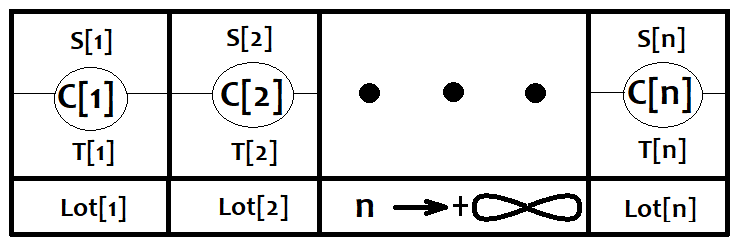• M[i]=(PT[i]*TP-PS[i]*SL)*Lot[i]*TickSize.

• M0=Sum(0,n)(PC[i]*M[i])

### 如何正确使用网格和马丁格尔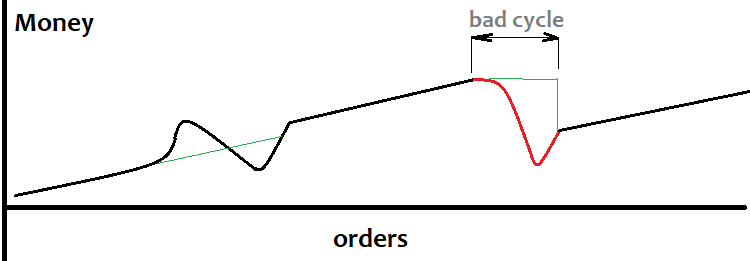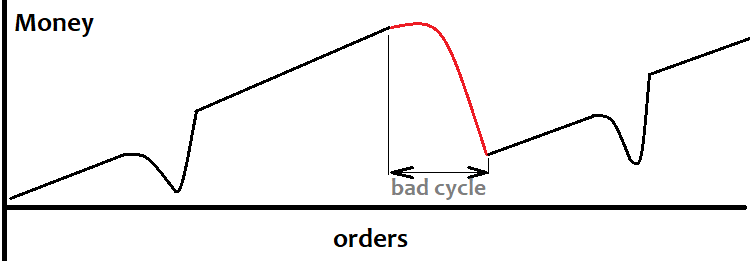## 量化课程

移动端课程